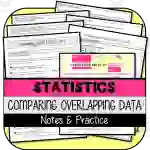Math > Statistics | Grade 7 | Activities

# Comparing Overlapping Data NOTES & PRACTICEAttributes
Subject

Statistics

Types

Activities

File

PDF

Editable
No### Comparing Overlapping Data NOTES & PRACTICE

This is an essential teaching resource aimed at 7th grade educators, focusing on making statistics learning enjoyable and practical, particularly in data handling. The key mathematical concepts taught include center measures such as mean, median, mode, and variability measures like range, IQR, MAD.

The resource comprises two main parts:

• 'Comparing Overlapping Data NOTES & PRACTICE': Enabling students to create informal comparisons of similar datasets both visually and through calculations. This part also encourages learners to compare the means of similar datasets within relation to their common variability (MAD).
• 'Comparing Overlapping Data TEST PRACTICE': This contains ten multiple-choice questions offered in two formats; a printable PDF or digitally via Google Forms allowing flexibility for teachers.

A unique aspect of this resource is the strong advice against calculator use especially during state tests - reinforcing practical arithmetic skills along with enhancing students' statistical abilities.

#### Versatility in Usage

The versatility with which this teaching aid can be leveraged involves:
• Whole class instruction where everyone engages collectively on practice questions.
• Smaller groups working collaboratively.
• Individual homework assignments encouraging independent thinking.
• Remote learning situations given its Google Forms compatibility.
Answer keys are provided for teacher convenience.

### Prior Knowledge Assumption

This resource does assume that learners are proficient in reading dot plots, box plots and histograms along with calculating center and variability measures.

If you're an educator focused on enhancing student outcomes while ensuring your own convenience - Comparing Overlapping Data NOTES & PRACTICE is a well-rounded tool aligned perfectly with the 7th grade math Statistics & Probability standards such as CCSS.MATH.CONTENT.7.SP.B.3/4.

## What's Included

This resources contains the following items:

1) Comparing Overlapping Data NOTES & PRACTICE:

· Informally compare similar data sets visually, giving generalizations about each set

· Informally compare similar data sets by calculating measures of center & variability to support the comparison statements

· Compare the means of similar data sets in relation to their common variability (MAD)

2) Comparing Overlapping Data TEST PRACTICE:

10 Multiple choice questions in two formats:

o Printable

CALCULATOR USE RECOMMENDED (especially if students are allowed to use calculators on state tests).

NOTE: This resource assumes students are proficient in reading dot plots, box plots, and histograms, as well as calculating measures of center (mean, median, mode) and measures of variability (range, IQR, MAD).

## Resource Tags

statistics data handling center measures variability measures comparative inferences

### you may also like...

Check out these other great products